# 2019 Fantasy Football Player RankingsDeveloping accurate fantasy football player rankings is a key component of creating reliable fantasy football cheat sheets, and as we know your cheat sheet could make or break your fantasy football draft. In modern fantasy football leagues, almost any football position can be added to your fantasy league scoring configuration (even defensive players).

Regardless of your league’s configuration, you’ll need to be sure to have accurate NFL player rankings for each position that is required to complete your fantasy roster. Otherwise, how would you know how to make picks for your fantasy football draft boards as the season approachs? Here are some factors we consider when developing our fantasy football player rankings:

• Statistics- Obviously the stats from the previous season are most critical but as the season progresses current statistics become more relevant.
• Fantasy Point Output- Understand how each player’s statistics translate to point output based on your fantasy league configuration. Just as statistic/point-values vary from league to league, a player's ranking will likewise rise or fall based on league settings.
• Games Played- The number of games a player actually played in during the previous season is an oft-forgotten statistic. Would you rather draft a player that put up 80 fantasy points in 16 games, or a player who put up 60 fantasy points in 8 games? This is why FPPG output is a key statistic to follow (and also why it is integrated into all of our sheets by default).
• Team- The team a player plays for should definitely factor into their ranking, especially if the player has changed teams. Those teams with high-powered offenses will have players who put up more fantasy points, so remember to factor in the team when you configure your rankings.
• Experience- The factor that experience plays in your NFL player rankings is heavily dependent on the position being analyzed; a productive kicker will have a much longer career than a productive running back. Compare each player’s stats to their experience to ensure they are not on the downside of their career.
• Position on Depth Chart- As training camp battles progress, a clearer picture of each team’s depth chart should arise. Know which players have cemented a starting job and which players are still battling to move up the depth chart.
• Injury Concerns– Football players are constantly battling injuries, but some injuries are worse than others. Those players with injury concerns should slide down your player rankings; how far they slide will depend on the severity (and sometimes the frequency) of their injury.

There are many different factors to consider when configuring your 2019 NFL player rankings. The key to success is examining the relevant factors and properly applying them to the position and player being analyzed. We hope that the rankings provided will help you create reliable and accurate fantasy football cheat sheets in preparation for your 2019 fantasy football draft. Below are our top 5 players at each position named in the standard fantasy football scoring system. To view all rankings for a particular position please click the links above.

## Top 10 Quarterbacks

(view all)
1Patrick Mahomes #13
 KC Chiefs, 1 years exp , Bye 12
GAM=16, PAYD=5097, PATD=50, RUCA=60, RUYD=272, RUTD=2, INT=12, FUM=2, FPPG=32.2, TFP=515
2Aaron Rodgers #12
 GB Packers, 14 years exp , Bye 11
GAM=16, PAYD=4442, PATD=25, RUCA=43, RUYD=269, RUTD=2, INT=2, FUM=3, FPPG=22.3, TFP=357
3Deshaun Watson #13
 HOU Texans, 2 years exp , Bye 10
GAM=16, PAYD=4165, PATD=26, RUCA=99, RUYD=551, RUTD=5, INT=9, FUM=3, FPPG=24, TFP=384
4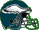Carson Wentz #12
 PHI Eagles, 3 years exp , Bye 10
GAM=11, PAYD=3074, PATD=21, RUCA=34, RUYD=93, RUTD=0, INT=7, FUM=6, FPPG=21.1, TFP=232
5Baker Mayfield #13
 CLE Browns, 1 years exp , Bye 7
GAM=14, PAYD=3725, PATD=27, RUCA=39, RUYD=131, RUTD=0, INT=14, FUM=3, FPPG=20.7, TFP=290
6Ben Roethlisberger #7
 PIT Steelers, 15 years exp , Bye 7
GAM=16, PAYD=5129, PATD=34, RUCA=31, RUYD=98, RUTD=3, INT=16, FUM=2, FPPG=25.1, TFP=401
7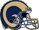Jared Goff #12
 LAR Rams, 3 years exp , Bye 9
GAM=16, PAYD=4688, PATD=32, RUCA=43, RUYD=108, RUTD=2, INT=12, FUM=5, FPPG=23, TFP=368
8Cam Newton #1
 CAR Panthers, 8 years exp , Bye 7
GAM=14, PAYD=3395, PATD=24, RUCA=101, RUYD=488, RUTD=4, INT=13, FUM=0, FPPG=23.3, TFP=327
9Matt Ryan #2
 ATL Falcons, 11 years exp , Bye 9
GAM=16, PAYD=4924, PATD=35, RUCA=33, RUYD=125, RUTD=3, INT=7, FUM=5, FPPG=25.8, TFP=413
10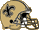Drew Brees #9
 NO Saints, 18 years exp , Bye 9
GAM=15, PAYD=3992, PATD=32, RUCA=31, RUYD=22, RUTD=4, INT=5, FUM=1, FPPG=24.4, TFP=366

## Top 10 Running Backs

(view all)
1Saquon Barkley #0
 NYG Giants, 1 years exp , Bye 11
GAM=16, RUCA=261, RUYD=1307, RUTD=11, RETR=121, RECP=91, REYD=721, RETD=4, FUM=0, FPPG=18.3, TFP=293
2Alvin Kamara #34
 NO Saints, 2 years exp , Bye 9
GAM=15, RUCA=194, RUYD=883, RUTD=14, RETR=105, RECP=81, REYD=709, RETD=4, FUM=0, FPPG=17.8, TFP=267
3Ezekiel Elliott #34
 DAL Cowboys, 3 years exp , Bye 8
GAM=15, RUCA=304, RUYD=1434, RUTD=6, RETR=95, RECP=77, REYD=567, RETD=3, FUM=1, FPPG=16.8, TFP=252
4Christian McCaffrey #34
 CAR Panthers, 2 years exp , Bye 7
GAM=16, RUCA=219, RUYD=1098, RUTD=7, RETR=124, RECP=107, REYD=867, RETD=6, FUM=1, FPPG=17, TFP=272
5James Conner #34
 PIT Steelers, 2 years exp , Bye 7
GAM=13, RUCA=215, RUYD=973, RUTD=12, RETR=71, RECP=55, REYD=497, RETD=1, FUM=2, FPPG=17, TFP=221
6Nick Chubb #34
 CLE Browns, 1 years exp , Bye 7
GAM=16, RUCA=192, RUYD=996, RUTD=8, RETR=29, RECP=20, REYD=149, RETD=2, FUM=0, FPPG=10.9, TFP=174
7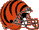Joe Mixon #34
 CIN Bengals, 2 years exp , Bye 9
GAM=14, RUCA=237, RUYD=1168, RUTD=8, RETR=55, RECP=43, REYD=296, RETD=1, FUM=0, FPPG=14.3, TFP=200
8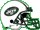Le'Veon Bell #26
 NYJ Jets, 5 years exp , Bye 4
9Leonard Fournette #23
 JAC Jaguars, 2 years exp , Bye 10
GAM=8, RUCA=133, RUYD=439, RUTD=5, RETR=26, RECP=22, REYD=185, RETD=1, FUM=0, FPPG=12.3, TFP=98
10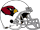David Johnson #31
 ARI Cardinals, 4 years exp , Bye 12
GAM=16, RUCA=258, RUYD=940, RUTD=7, RETR=76, RECP=50, REYD=446, RETD=3, FUM=2, FPPG=12.2, TFP=195

(view all)
1DeAndre Hopkins #10
 HOU Texans, 6 years exp , Bye 10
GAM=16, RETR=163, RECP=115, REYD=1572, RETD=11, RUYD=-7, RUTD=0, FUM=2, FPPG=13.7, TFP=218
2GB Packers, 5 years exp , Bye 11
GAM=15, RETR=169, RECP=111, REYD=1386, RETD=13, RUYD=0, RUTD=0, FUM=0, FPPG=14.4, TFP=217
3Tyreek Hill #80
 KC Chiefs, 3 years exp , Bye 12
GAM=16, RETR=137, RECP=87, REYD=1479, RETD=12, RUYD=151, RUTD=1, FUM=0, FPPG=15.1, TFP=241
4Juju Smith-Schuster #80
 PIT Steelers, 2 years exp , Bye 7
GAM=16, RETR=166, RECP=111, REYD=1426, RETD=7, RUYD=13, RUTD=0, FUM=1, FPPG=11.5, TFP=184
5Michael Thomas #80
 NO Saints, 3 years exp , Bye 9
GAM=16, RETR=147, RECP=125, REYD=1405, RETD=9, RUYD=0, RUTD=0, FUM=2, FPPG=11.9, TFP=190
6Julio Jones #11
 ATL Falcons, 8 years exp , Bye 9
GAM=16, RETR=170, RECP=113, REYD=1677, RETD=8, RUYD=12, RUTD=0, FUM=2, FPPG=13.3, TFP=213
7Odell Beckham #13
 CLE Browns, 4 years exp , Bye 7
GAM=12, RETR=124, RECP=77, REYD=1052, RETD=6, RUYD=19, RUTD=0, FUM=1, FPPG=11.8, TFP=141
8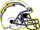Keenan Allen #13
 LAC Chargers, 6 years exp , Bye 12
GAM=15, RETR=136, RECP=97, REYD=1196, RETD=6, RUYD=75, RUTD=0, FUM=1, FPPG=10.7, TFP=161
9MIN Vikings, 5 years exp , Bye 12
GAM=16, RETR=155, RECP=113, REYD=1373, RETD=9, RUYD=30, RUTD=0, FUM=1, FPPG=12, TFP=192
10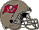Mike Evans #13
 TB Buccaneers, 5 years exp , Bye 7
GAM=16, RETR=138, RECP=86, REYD=1524, RETD=8, RUYD=0, RUTD=0, FUM=1, FPPG=12.4, TFP=198

## Top 10 Tight Ends

(view all)
1Travis Kelce #87
 KC Chiefs, 6 years exp , Bye 12
GAM=16, RETR=150, RECP=103, REYD=1336, RETD=10, RUYD=0, RUTD=0, FUM=1, FPPG=12, TFP=192
2Zach Ertz #86
 PHI Eagles, 6 years exp , Bye 10
GAM=16, RETR=156, RECP=116, REYD=1163, RETD=8, RUYD=0, RUTD=0, FUM=0, FPPG=10.3, TFP=164
3George Kittle #85
 SF 49ers, 2 years exp , Bye 4
GAM=16, RETR=136, RECP=88, REYD=1377, RETD=5, RUYD=10, RUTD=0, FUM=0, FPPG=10.5, TFP=169
4Evan Engram #85
 NYG Giants, 2 years exp , Bye 11
GAM=11, RETR=64, RECP=45, REYD=577, RETD=3, RUYD=36, RUTD=0, FUM=0, FPPG=7.2, TFP=79
5O.J. Howard #85
 TB Buccaneers, 2 years exp , Bye 7
GAM=9, RETR=45, RECP=34, REYD=565, RETD=5, RUYD=0, RUTD=0, FUM=0, FPPG=9.6, TFP=86
6Hunter Henry #0
 LAC Chargers, 3 years exp , Bye 12
7Jared Cook #89
 NO Saints, 9 years exp , Bye 9
GAM=16, RETR=101, RECP=68, REYD=896, RETD=6, RUYD=0, RUTD=0, FUM=0, FPPG=7.8, TFP=126
8Delanie Walker #82
 TEN Titans, 13 years exp , Bye 11
GAM=1, RETR=7, RECP=4, REYD=52, RETD=0, RUYD=0, RUTD=0, FUM=0, FPPG=5.2, TFP=5
9Mark Andrews #85
 BAL Ravens, 1 years exp , Bye 8
GAM=15, RETR=48, RECP=34, REYD=552, RETD=3, RUYD=0, RUTD=0, FUM=0, FPPG=4.9, TFP=73
10David Njoku #85
 CLE Browns, 2 years exp , Bye 7
GAM=15, RETR=89, RECP=56, REYD=639, RETD=4, RUYD=0, RUTD=0, FUM=0, FPPG=5.9, TFP=88

## Top 10 Kickers

(view all)
1Greg Zuerlein #4
 LAR Rams, 7 years exp , Bye 9
GAM=11, MAFG=27, MIFG=4, MAXP=35, MIXP=1, FPPG=9.4, TFP=103
2Will Lutz #3
 NO Saints, 3 years exp , Bye 9
GAM=16, MAFG=28, MIFG=2, MAXP=52, MIXP=1, FPPG=8.1, TFP=129
3Stephen Gostkowski #3
 NE Patriots, 13 years exp , Bye 10
GAM=16, MAFG=27, MIFG=5, MAXP=49, MIXP=1, FPPG=7.1, TFP=114
4Justin Tucker #9
 BAL Ravens, 7 years exp , Bye 8
GAM=16, MAFG=35, MIFG=4, MAXP=36, MIXP=1, FPPG=8, TFP=128
5Harrison Butker #8
 KC Chiefs, 2 years exp , Bye 12
GAM=16, MAFG=24, MIFG=3, MAXP=65, MIXP=4, FPPG=7.8, TFP=124
6Robbie Gould #9
 SF 49ers, 14 years exp , Bye 4
GAM=16, MAFG=33, MIFG=1, MAXP=27, MIXP=2, FPPG=7.6, TFP=121
7Ka'imi Fairbairn #7
 HOU Texans, 3 years exp , Bye 10
GAM=16, MAFG=37, MIFG=5, MAXP=39, MIXP=2, FPPG=8.3, TFP=133
8Jake Elliott #8
 PHI Eagles, 2 years exp , Bye 10
GAM=16, MAFG=26, MIFG=5, MAXP=33, MIXP=2, FPPG=5.9, TFP=94
9Mason Crosby #2
 GB Packers, 12 years exp , Bye 11
GAM=15, MAFG=30, MIFG=7, MAXP=34, MIXP=2, FPPG=6.7, TFP=101
10IND Colts, 23 years exp , Bye 6
GAM=15, MAFG=23, MIFG=4, MAXP=44, MIXP=3, FPPG=6.5, TFP=98

## Top 10 Defenses/Special Teams

(view all)
1Chicago Bears
 Bye 6
GAM=16, FREC=9, INT=27, SACK=50, DTD=6, PA=283, FPPG=10.1, TFP=162
2Jacksonville Jaguars
 Bye 10
GAM=16, FREC=6, INT=11, SACK=37, DTD=2, PA=316, FPPG=5.7, TFP=91
3Los Angeles Rams
 Bye 9
GAM=16, FREC=12, INT=18, SACK=41, DTD=4, PA=384, FPPG=8.3, TFP=133
4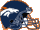Denver Broncos
 Bye 10
GAM=16, FREC=11, INT=17, SACK=44, DTD=2, PA=349, FPPG=7, TFP=112
5San Diego Chargers
 Bye 12
GAM=16, FREC=7, INT=13, SACK=38, DTD=2, PA=329, FPPG=5.6, TFP=90
6Minnesota Vikings
 Bye 12
GAM=16, FREC=8, INT=12, SACK=50, DTD=3, PA=341, FPPG=6.8, TFP=108
7New England Patriots
 Bye 10
GAM=16, FREC=10, INT=18, SACK=30, DTD=2, PA=325, FPPG=6.1, TFP=98
8New Orleans Saints
 Bye 9
GAM=16, FREC=12, INT=12, SACK=49, DTD=1, PA=353, FPPG=6.4, TFP=103
9Baltimore Ravens
 Bye 8
GAM=16, FREC=5, INT=12, SACK=43, DTD=3, PA=287, FPPG=5.9, TFP=95
10Dallas Cowboys
 Bye 8
GAM=16, FREC=11, INT=9, SACK=39, DTD=1, PA=324, FPPG=5.3, TFP=85

Miscellaneous
Social Sites
Legal

We are a participant in the Amazon Services LLC Associates Program, an affiliate advertising program designed to provide a means for us to earn fees by linking to Amazon.com and affiliated sites.